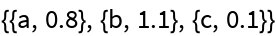#Function Repository Resource:

# MetaTally

Combine multiple tally results

Contributed by: David Gathercole
 ResourceFunction["MetaTally"][{t1,t2,…}] combines the counts of multiple tally results ti. ResourceFunction["MetaTally"][{t1,t2,…},f] applies f to the list of counts of each given element, where by default these lists are totaled.

## Details and Options

By way of an extension, ResourceFunction["MetaTally"] combines all but the first element of the second-level lists.
The default aggregation function is Total.

## Examples

### Basic Examples

MetaTally combines multiple tallies:

 In:=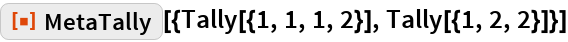Out=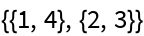Analogous to the Tally of the combined lists:

 In:=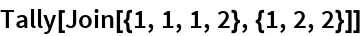Out=Allowing work with intermediate tallies:

 In:=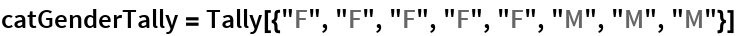Out=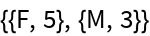In:=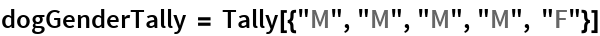Out=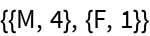In:=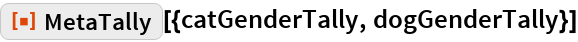Out=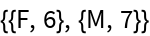### Scope

The argument lists are compared on their first elements:

 In:=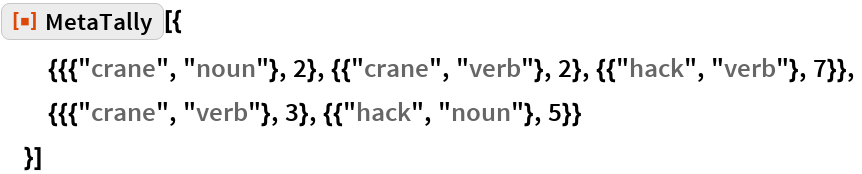Out=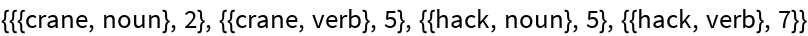The aggregation is performed on the rest of the elements:

 In:=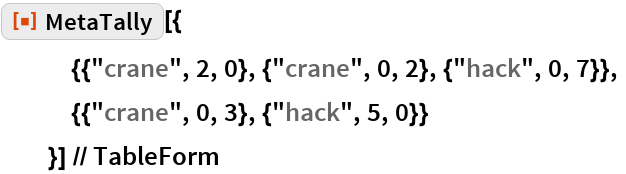Out=The function for aggregation can be specified:

 In:=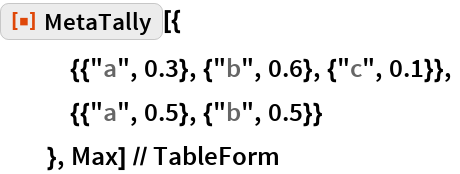Out=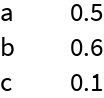In:=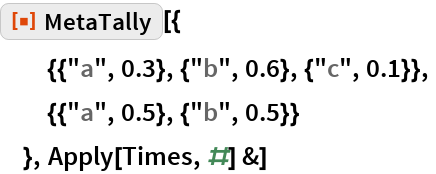Out=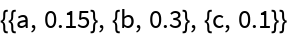### Properties and Relations

The default aggregation function is Total:

 In:=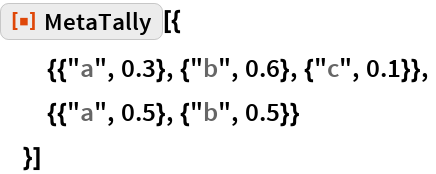Out=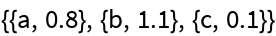In:=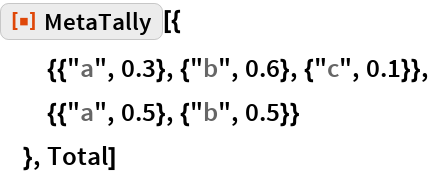Out=